# Experience of implementing LINEST in anaplanHello colleagues.
At the moment I am implementing statistical data analysis in anaplan, the analysis methodology was developed in an excel.

In excel, the LINEST function was used to search for the four unknowns using the least squares method.
I did not find an analogue of this function and I do not imagine how to obtain the necessary values.
Did you have experience using the function LINEST in Anaplan?

How did you solve this problem?

Ilya_Zhivov

Tagged:

•May be i am missing something here but it sounds like a simple regression to me based on what you highlighted . Below i have plotted some numbers in excel and used Linest function to show regression results

Here is First screen shot where you can see data i made based on scenario you just explained

We are predicting sales based on weeks where Z a constant to mark b as 0 in case its 0 , unsure what is d?

you will notice on the graph that i have a simple regression line showing y=ax+ b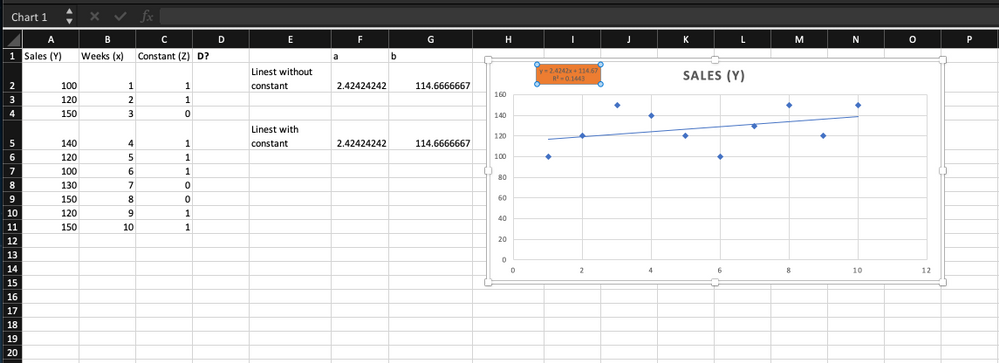Now i am doing the same workings using linest function without constant i.e z set to nothing . Notice how my a and b matches regression line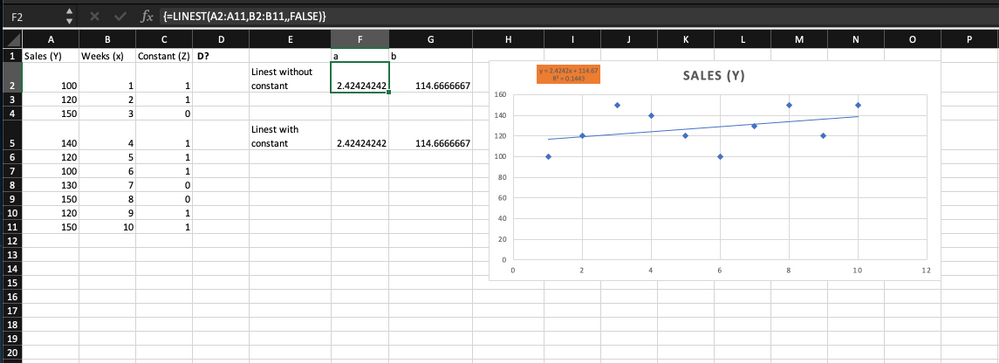Here is a screen shot of linest function with constant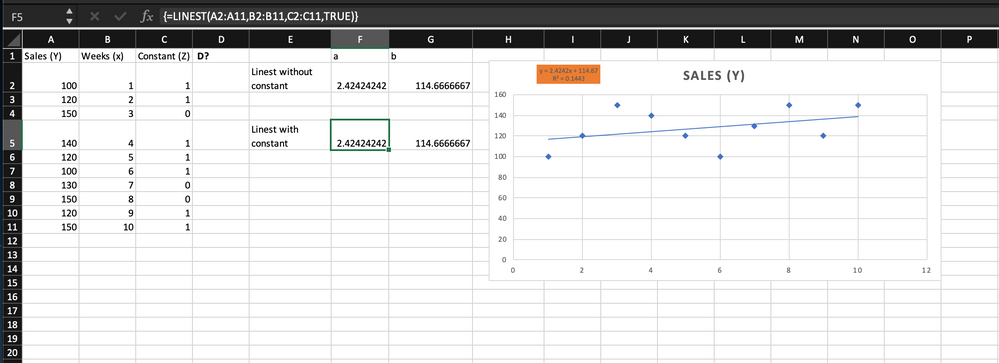again values are the same as regression line .

I showed you earlier regression is achievable in anaplan . So unless we know what data you are looking at it will be hard to know exactly what the issue is . Perhaps you could share something like what i have done above to know more about whats going on at your end .

I am sure we can implement simple as well as multi(quadratic) regression in anaplan which is what i think linest function does

Hope this helps

•Hi @Ravil

There's no straightforward formulation to solve linear regression, whereas there's a functionality for linear programming. Check this https://help.anaplan.com/anapedia/Content/Modeling/Optimizer/anaplan-optimizer.htm for better understanding of the same.

Hope this helps.

Regards,

Pavan

•Hi

Unsure about linest function but recently i implemented a trend line or simple linear regression for a client . Below are some screen shots of how i did it .

Green line is the trend line or simple regression forecast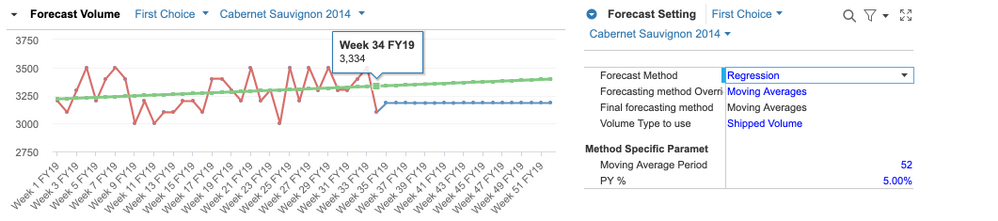Calculated intercept a and slope b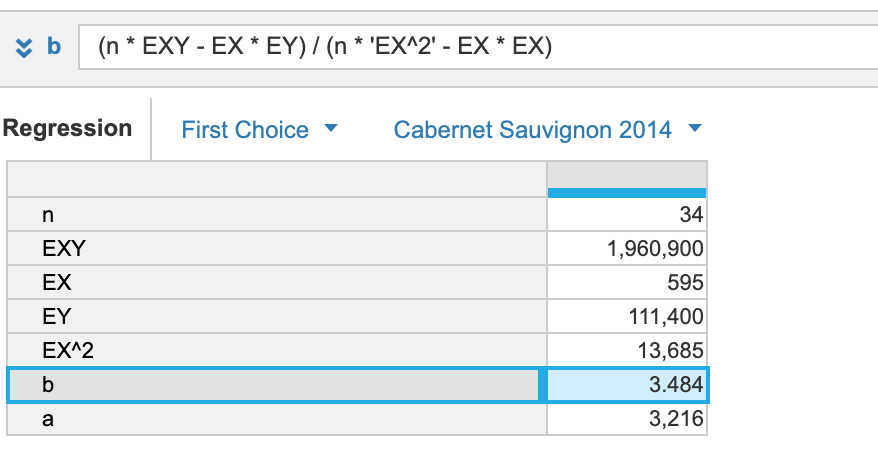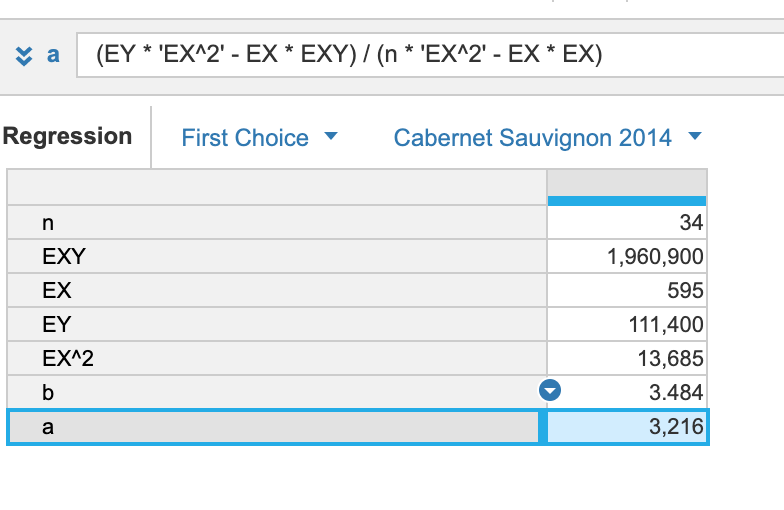calculated

y = a + bx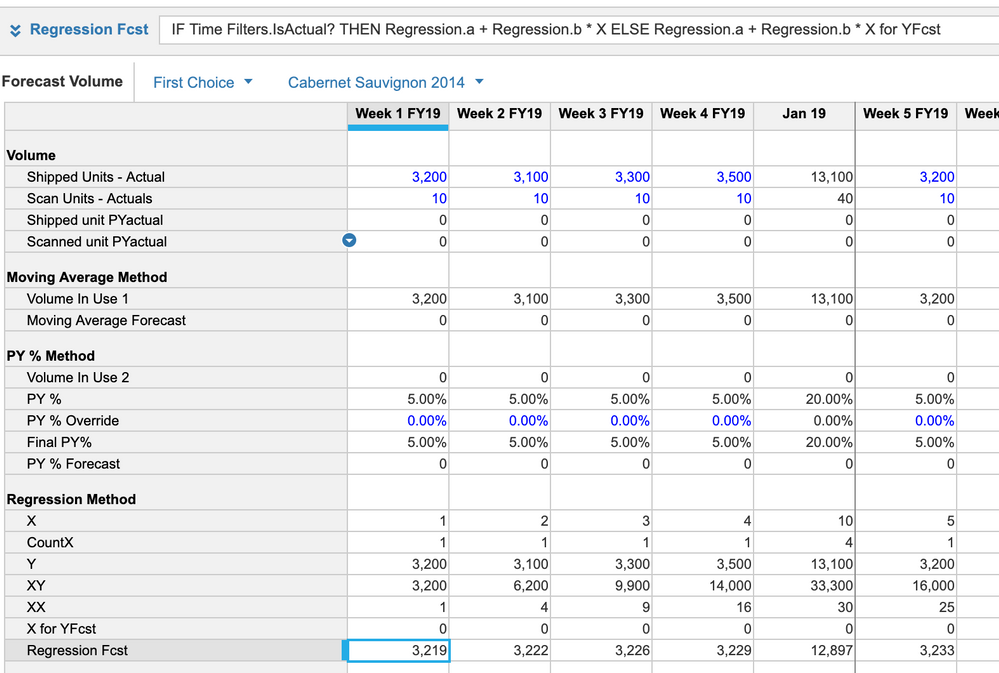I guess linest and regression should return the same trend line . I could be wrong

hope this helps

•Thank you @karank, but I think my task is a little more difficult, the function looks like this: Y=ax^2+bx+cz+d

Where to find: a, b, c, d

•Hi

What is cz ? or do you mean Y=ax^2 + bx + cx + d

if above is the case then you probably have 3 variable multiple regression and d is the error coefficient and your data when plotted looks like a parabola ? (I am Just assuming things)

It will be helpful if you can plot your data sample on a graph and show here .

•No, i mean Y=ax^2 + bx + cz + d

y - my data, let it be sales

х - timeline, let it be the week number

z - is it one if there was a holiday this week, or zero if it is a regular week

a, b, c, d - unknowns computed by function

•Hey.
Yes, of course, it is possible in Anaplan.
We found a way to repeat the lineest function through the matrix system in anaplan, this solution weighs only 5MB and takes several modules and reference books, but it works very quickly.

I'm not sure if I can describe the method in detail right now here.

I'll try to do this later.